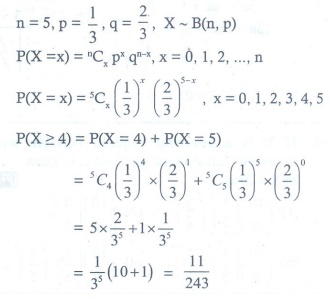# Chapter 11: Probability Distributions - Online Test

Q1.

Let x be random variable with probability density function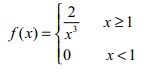Which of the following statement is correct?
Explaination / Solution: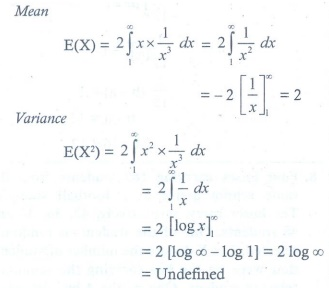Q2.

A rod of length 2l is broken into two pieces at random. The probability density function of the shorter of the two pieces is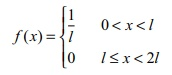The mean and variance of the shorter of the two pieces are respectively

Explaination / Solution: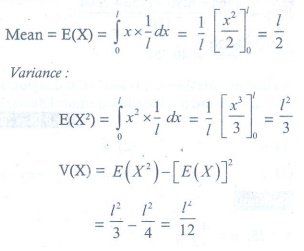Q3. Consider a game where the player tosses a six-sided fair die. If the face that comes up is 6, the player wins 36, otherwise he loses k 2 , where k is the face that comes up k = {1, 2, 3, 4, 5}. The expected amount to win at this game in is
Explaination / Solution: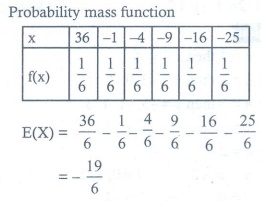Q4. A pair of dice numbered 1, 2, 3, 4, 5, 6 of a six-sided die and 1, 2, 3, 4 of a four-sided die is rolled and the sum is determined. Let the random variable X denote this sum. Then the number of elements in the inverse image of 7 is
Explaination / Solution: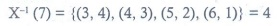Q5. A random variable X has binomial distribution with n = 25 and p = 0.8 then standard deviation of X is
Explaination / Solution: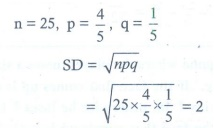Q6. Let X represent the difference between the number of heads and the number of tails obtained when a coin is tossed n times. Then the possible values of X are
Explaination / Solution: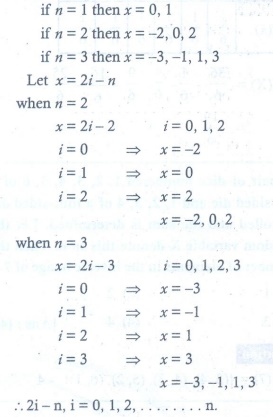Q7. If the function f (x) = 1/12 for a < x < b , represents a probability density function of a continuous random variable X, then which of the following cannot be the value of a and b?
Explaination / Solution: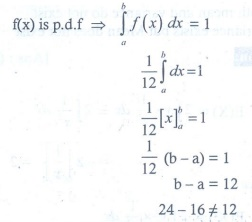Q8.

Four buses carrying 160 students from the same school arrive at a football stadium. The buses carry, respectively, 42, 36, 34, and 48 students. One of the students is randomly selected. Let X denote the number of students that were on the bus carrying the randomly selected student. One of the 4 bus drivers is also randomly selected. Let Y denote the number of students on that bus.

Then E(X) and E(Y) respectively are
Explaination / Solution: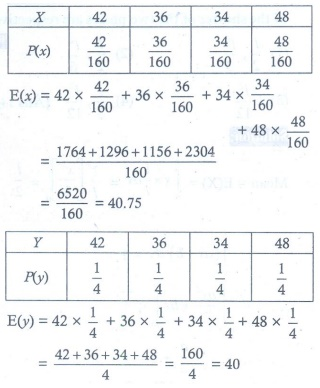Q9. Two coins are to be flipped. The first coin will land on heads with probability 0.6, the second with Probability 0.5. Assume that the results of the flips are independent, and let X equal the total number of heads that result. The value of E(X) is
Explaination / Solution: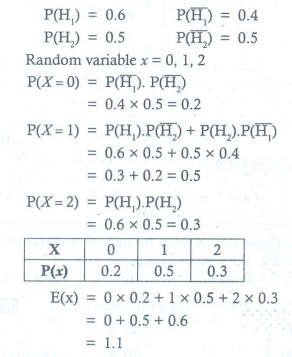Q10. On a multiple-choice exam with 3 possible destructives for each of the 5 questions, the probability that a student will get 4 or more correct answers just by guessing is
Explaination / Solution: